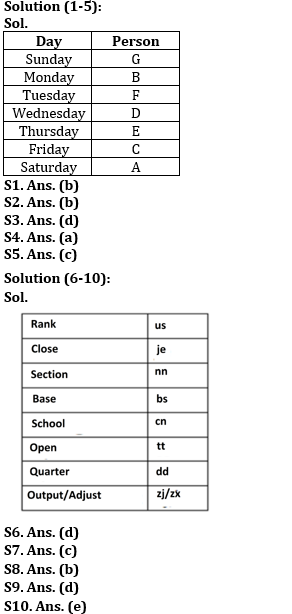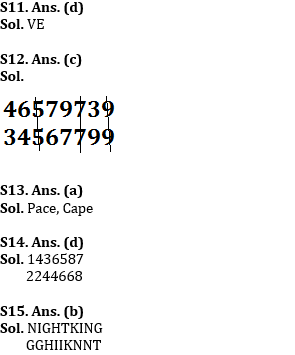Latest Banking jobs   »   Reasoning Ability Quiz For RBI Grade...

# Reasoning Ability Quiz For RBI Grade B/ ECGC PO/ SIDBI Grade A Prelims 2022- 23rd April

Directions (1-5): Study the following information carefully and answer the given questions.
Seven persons A, B, C, D, E, F and G have exam on seven different days of the same week starting from Sunday to Saturday.
More than three persons has exam before E. G has exam before D and only two people has exam between them. C has exam immediate before A and none of them has exam on Monday. B has exam immediate before F. The number of persons has exam between F and G is less than number of persons has exam between D and A.

Q1. Who has exam on Monday?
(a) A
(b) B
(c) C
(d) D
(e) None of these

Q2. Who has exam just before F?
(a) A
(b) B
(c) C
(d) D
(e) None of these

Q3. How many persons have exam in between B and C?
(a) More than three
(b) One
(c) Two
(d) Three
(e) No one

Q4. Who has exam on Saturday?
(a) A
(b) B
(c) C
(d) D
(e) None of these

Q5. Who has exam just after E?
(a) A
(b) B
(c) C
(d) D
(e) None of these

Directions (6-10): Study the following information carefully and answer the questions accordingly.
In a certain code language,
‘School quarter section base’ is coded as ‘dd bs nn cn’
‘Rank base output adjust’ is coded as ‘us bs zj zx’
‘Rank close section base’ is coded as ‘us je nn bs’
‘School open close section’ is coded as ‘nn cn tt je’

Q6. What is the code for ‘open’?
(a) nn
(b) je
(c) cn
(d) tt
(e) None of these

Q7. Which of the following is coded as ‘dd’?
(a) School
(b) Base
(c) Quarter
(d) Section
(e) None of these

Q8. Which of the following is the possible code for ‘Base Manners’?
(a) nn du
(b) gn bs
(c) vb gj
(d) cn nn
(d) None of these

Q9. What is the code for ‘open Close’?
(a) je cn
(b) tt bs
(c) nn us
(d) je tt
(e) None of these

Q10. Which of the following is coded as ‘zx’?
(a) Base
(b) Output
(d) Rank
(e) Either B or C

Q11. What should come in place of question mark (?) in the following series based on the above arrangement?
ZA   YB   XC   WD   ?
(a) EU
(b) LO
(c) LP
(d) VE
(e) None of these

Q12. How many numbers will be remained at the same position in the given number “46579739” when they are arranged in the ascending order from left to right?
(a) One
(b) Two
(c) Three
(d) More than three
(e) None of these.

Q13. If it is possible to make only one meaningful word with the 1st ,2nd ,4th and 7th letters of the word ‘CAREERPOWER’ which would be the third letter of the word from the right? If more than one such word can be formed give ‘Y’ as the answer. If no such word can be formed, give ‘Z’ as your answer.
(a) Y
(b) P
(c) C
(d) Z
(e) A

Q14. If 1 is added to each odd digit and 2 is subtracted from each even digit in the number 1436587, then how many digits will appear twice in the new number thus formed?
(a) Only 8
(b) Only 8 and 6
(c) 8, 6 and 4
(d) 2, 4 and 6
(e) None of these

Q15. How many letters will be remaining at the same position in the word ‘NIGHTKING’ when letters arranged according to alphabetical order from left to right?
(a) One
(b) Two
(c) Three
(d) More than Three
(e) None

Solutions